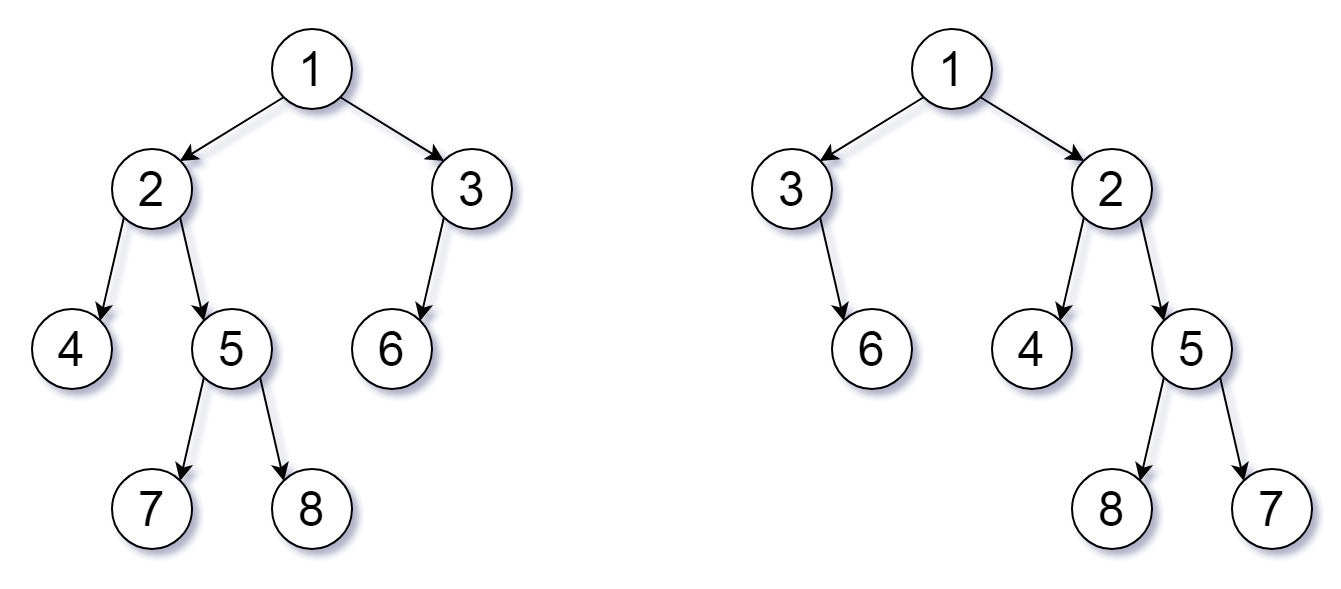## 算法分析课程博客分享 13

### 951. 翻转等价二叉树(Flip Equivalent Binary Trees)• 每棵树最多有 100 个节点。
• 每棵树中的每个值都是唯一的、在 [0, 99] 范围内的整数。

``````bool flipEquiv(TreeNode* root1, TreeNode* root2) {
if (root1 == NULL && root2 == NULL)
return true;
if (root1 == NULL || root2 == NULL)
return false;
if (root1->val != root2->val)
return false;

return (flipEquiv(root1->left, root2->right) && flipEquiv(root1->right, root2->left)) ||
(flipEquiv(root1->left, root2->left) && flipEquiv(root1->right, root2->right));
}
``````

### 942. 增减字符串匹配(DI String Match)

• 1 <= S.length <= 1000
• S 只包含字符 “I” 或 “D”。

``````vector<int> diStringMatch(string S) {
vector<int> res;
int I = 0, D = S.length();
for (char c : S) {
if (c == 'I')
res.push_back(I++);
else
res.push_back(D--);
}
res.push_back(S.back() == 'I' ? I : D);

return res;
}
``````

### 944. 删列造序(Delete Columns to Make Sorted)

• 1 <= A.length <= 100
• 1 <= A[i].length <= 1000

``````int minDeletionSize(vector<string>& A) {
int res = 0;
for (int i = 0; i < A.size(); ++i) {
for (int j = 1; j < A.size(); ++j) {
if (A[j][i] < A[j-1][i]) {
++res;
break;
}
}
}

return res;
}
``````